# All commands, alphabetic order; letter L

## \l (constant)

The \l command produces an l with a stroke, (Unicode U+142, ł). (See also \AA or extended latin characters).

## \L (constant)

The \L command produces a capital L with a stroke, (Unicode U+141, Ł). (see also extended latin characters).

## \label

The \label command can be used to put a label somewhere. The anchor can be a sectioning command, see the description of the \part command. It can also be a figure, a table, a math formula, a footnote, an item in a list. Here are some cases.

\paragraph{A}
\label{pa}
\ref{pa} \ref{pb} \ref{pc} \ref{pd} \ref{pe} \ref{pf}
\paragraph{B}
\label{pb}
\begin{equation}x \label{pc} \end{equation}
\begin{figure} \includegraphics{x}\label{pd} \end{figure}
\begin{itemize} \item a \label{pe}\item b \label{pf} \end{itemize}


The XML code is the following

<div3 id-text='0.0.0.1 id='uid19'><head>A</head>
<p><ref target='uid19'/> <ref target='uid20'/> <ref target='uid21'/> <ref target='uid22'/>
<ref target='uid23'/> <ref target='uid24'/></p>
</div3>
<div3 id-text='0.0.0.2' id='uid20'><head>B</head>
<formula  id-text='1' id='uid21' type='display'><math xmlns='http://www.w3.org/1998/Math/MathML'><mi>x</mi>[/itex]</formula>
<figure file='x' id-text='1' id='uid22'></figure>
<list type='simple'>
<item id-text='1' id='uid23'><p>a </p>
</item>
<item id-text='1' id='uid24'><p>b  </p>
</item></list>
...
</div3>


## \labelitemi, \labelitemii, \labelitemiii, \labelitemiv

The default value of an item label (case where \item is given without optional argument) is defined by \labelitemi, a bullet. In the case of nested lists, it is the value of \labelitemii, \labelitemiii, or \labelitemiv. Not used by Tralics.

## \labelsep (rigid length)

Space between a label and the text that follows in an item. Unused by Tralics.

## \labelwidth (rigid length)

The normal width of a box that contains the label in an item. Unused by Tralics.

## \lambda (math symbol)

The \lambda command is valid only in math mode. It generates a Greek letter: <mi>&lambda;</mi> (Unicode U+3BB, λ). (See description of the \alpha command).

## \Lambda (math symbol)

The \Lambda command is valid only in math mode. It generates an uppercase Greek letter: <mi>&Lambda;</mi> (Unicode U+39B, Λ). (See description of the \alpha command.)

## \land (math symbol)

The \land command (logical and) is equivalent to \wedge command: <mo>&wedge;</mo> (Unicode U+2227, ∧). (See also description of the \smallint command.)

## \langle (math symbol)

The \langle command is valid only in math mode. It generates a delimiter (left angle): <mo>&langle;</mo> (Unicode U+2329, 〈). (See description of the \vert command.)

## \language (internal integer)

When you say \language=79, the language number 79 is selected. Tralics knows the following languages: 0 for English, 1 for French, 2 for German, and 3 for the other one. More explanations should be given... (See scanint for details of argument scanning).

## \large

The \large command is a command that selects a large font. For an example of fonts, see \rm.

## \Large

The \Large command is a command that selects a large font. For an example of fonts, see \rm.

## \LARGE

The \LARGE command is a command that selects a large font. For an example of fonts, see \rm.

## \lastbox

In the case where the last item on the current list (XML tree) is a box, the \lastbox takes it off the stack and returns it. Otherwise, \lastbox returns an empty box. This does not work in math mode. Example

\def\dupbox{\setbox0=\lastbox \copy0 \box0 }
\xbox{foo}{ok} and \dupbox; \xbox{bar}{ok}\dupbox.


Translation.

<foo>ok</foo><p>and ; <bar>ok</bar><bar>ok</bar>.</p>


## \lastkern

The command \lastkern gives access to a read-only variable, that contains a dimension. The location pointed to is never modified by Tralics, so the \the\lastkern always expands to 0pt. On the other hand \lastkern=3pt provokes an error before reading the equals sign. See \lastpenalty below.

## \lastpenalty

According to TeX: You can't use \lastpenalty' in vertical mode. You can't use \lastpenalty' in horizontal mode. You can't use \lastpenalty' in math mode. In fact, you can only consult the value. If the last token on the current list is a penalty token, \the\lastpenalty returns the value; otherwise it returns 0. Since Tralics does not inserts penalties in (the equivalent of) its current list, the result is always the integer 0.

## \lastskip

The command \lastskip gives access to a read-only variable, that contains a glue. The location pointed to is never modified by Tralics, so the \the\lastskip always expands to 0pt. On the other hand \lastskip=3pt provokes an error before reading the equals sign.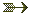See \lastpenalty above.

## \latex (from the html package)

Translation of \latex[foo]{bar} is foo.

## \latexhtml (from the html package)

Translation of \latex{foo}{bar} is foo.

## \latextohtml (from the html package)

This is the logo of the latex2html interpreter, defined as \textup{\LaTeX2\texttt{HTML}}.

## \LaTeX, \LaTeXe (constant)

The \LaTeX command translates to <LaTeX>, and \LaTeXe is the same as \LaTeX2$\epsilon$.

## latexonly (environment)

The environments latexonly and htmlonly are provided by the html package as alternatives, when converting a document to dvi or HTML. The first environment behaves like the identity, the second as a comment. Example


\def\ok{4} \leavevmode
\begin{rawxml}
&#x31;
\end{rawxml}
\begin{latexonly}\def\ok{2}\ok\end{latexonly}
\begin{htmlonly}
\def\ok{ ?
\end{htmlonly}
\begin{comment}
\def\ok{ ?
\end{comment}
\begin{rawhtml}
<p>\def\ok{ ?</p>
\end{rawhtml}
\begin{LaTeXonly}
\def\ok{ ?
\end{LaTeXonly}
\begin{xmlonly}\def\ok{3}\ok\end{xmlonly}
\ok


The translation should be equivalent to 1 2 3 4 (the digit one being written as &#x31;).

## LaTeXonly (Tralics environment)

The LaTeXonly environment is opposed to the xmlonly environment. Since the translator produces XML, the content of the LaTeXonly environment is ignored. This environment behaves like the comment environment.For an example, see the description of the latexonly environment.

## \lbrack

The \lbrack command is equivalent to (in fact, expands to) an opening bracket [.See also description of the \vert command.

## \lbrace (math symbol)

The \lbrace command is equivalent to an opening brace \{.See also description of the \vert command.

## \lccode

When you say \lccode13 12, you change the lowercase code of the character number 13 to the value 12. Both integers must be valid character codes. TeX uses this for its hyphenation algorithm: a letter is part of a word, only if its \lccode is not zero. On the other hand, the \lowercase command replaces, in the token list given as argument, every character X by the character Y, provided that \lccode X is Y, and Y is not zero; all other tokens are left unchanged.
A character code is an integer between 0 and N-1, where N=256 in TeX and in old versions of Tralics; it is now 65536. In Tralics, \lccode and \uccode are defined for all letters of iso-8859-1 (with, and without accents), these are characters with code less than 256 (for all other characters, \lccode and \uccode are zero).See scanint for details of how numbers are read. See \uppercase. for examples of use.

## \lceil (math symbol)

The \lceil (left ceiling) command is valid only in math mode. It can be used as a delimiter: <mo>&lceil;</mo>, (Unicode U+2308, ⌈). See also description of the \vert command.

## \ldots (math symbol)

The \ldots command is valid in math mode. It generates a miscellaneous symbol: <mo>...</mo>. Tralics recognizes the following miscellaneous symbols.

$\ldots \prime \exists \Diamond \top \bot \mho \cdots \forall \nabla \imath \flat \clubsuit \Re \vdots \infty \surd \jmath \natural \diamondsuit \Im \ddots \hbar \square \ell \sharp \heartsuit \angle \aleph \emptyset \triangle \neg \wp \spadesuit \partial$


The XML result is shown here. All these operators are from Table 8.7 of the Latex Companion. The \Box command is not yet implemented (but \square produces the same effect).(see also here).The \ldots command is also valid outside math mode, see the \qquad command.

## \le (math symbol)

The \le command is valid only in math mode. It generates a relation symbol: <mo>&le;</mo>. Tralics recognizes the following relations:

$\le \leq \succ \simeq \parallel \subseteq \sqsubset \doteq \ge \geq \sim \mid \subset \subseteq \ne \neq \frown \vdash \equiv \perp \ll \supset \cong \smile \in \dashv \models \preceq \gg \approx \Join \sqsubseteq \ni \prec \succeq \asymp \bowtie \sqsupseteq \propto$


The XML result is shown here. All these operators are from Table 8.5 of the Latex Companion.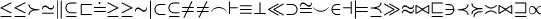(see also here).

## \leaders

The \leaders commands reads a box and names it leaders'. See \xleaders for details.

## \leadsto

The \leadsto command is valid only in math mode. It generates <mo>&#x21DD;</mo>, (Unicode U+21DD, ⇝).

## \leavevmode

The effect of this command is to leave vertical mode, and to enter horizontal mode. Thus, if the mode is vmode (typically, outside any paragraph), a new paragraph is started. This paragraph may be flushed left, flushed right, or centered; but if nothing special has been said, a noindent='true' slot is added to the attribute list of the <p> element.

## \left

This command is followed by a delim, then math mode material, then \right and delim; it is used inside a math formula. A group is started, the math mode material (the longest list, balanced wrt braces and groups, including \left/\right) is translated, the result is put in a <mfence> element, that has as attributes open' and close' the values of the first and last delimiter.

Here delim is a filler (optional space or \relax) followed by \delimiter 27-bit number or letter or otherchar (a character of catcode 11 or 12 whose 24-bit \delcode value will be used). From these numbers two pairs (a,f) and (b,g) consisting of a 8-bit character number and a 4-bit family number are created. These are used to construct a variable-size character. Note that commands like \overwithdelims also read two delim, so $a\abovewithdelims\relax.\relax..2ptb$ is a valid math formula translated by Tralics as $a\above.2ptb$

For instance \lbrace is defined as \delimiter"4266308 which gives a first pair: character 102 for family 2, and a second pair: character 8 in family 3. Now, font cmex10 (\texfont3 in PlainTeX) contains a character at position O10 (read: octal-10), that has a nextlarger at O156, that has a nextlarger at O32, that has a nextlarger at O50, that has a nextlarger at O70, that is defined to be a varchar top=O70, mid=O74, bot=O72 and rep=O76 (a varchar is a character of variable height, formed by a top, a rep, a mid, a rep and a bot; instead of a single rep, a variable number of reps can be used).

This mechanism is not implemented in Tralics. In particular \delimiter provokes an error and reads a number. You may use the following characters: ()[]|<> or the following commands:\rbrace \lbrace \{ \} \rangle \langle \rceil \lceil \rfloor \lfloor \vert \Vert. These are defined by PlainTeX. A dot means an empty delimiter.

You can also use \lmoustache \rmoustache \uparrow \downarrow \updownarraow \Uparrow \Downarrow \Updownarrow \backslash \lgroup \rgroup . These were not implemented in earlier Tralics versions: \arrowvert \Arrowvert \bracevert / \backslash.For an example, see the \vert command. Here is another example:

\def\kernel{\frac{x^1_2}{y^3_4}}
\def\test{
\left\lmoustache\left\uparrow\left\Uparrow\left\updownarrow\left\lgroup
\kernel
\right\rgroup\right\Updownarrow\right\Downarrow\right\downarrow\right\rmoustache}
$\test$
$\let\left\relax\let\right\relax \test$
$\def\kernel{\int_0^{\frac{x^1_2}{y^3_4}}} \test$


The translatiton is

<formula type='inline'>
<math xmlns='http://www.w3.org/1998/Math/MathML'>
<mfenced open='&lmoustache;' close='&rmoustache;'>
<mfenced open='&uparrow;' close='&downarrow;'>
<mfenced open='&Uparrow;' close='&Downarrow;'>
<mfenced open='&updownarrow;' close='&Updownarrow;'>
<mfenced open='&#x3014;' close='&#x3015;'>
<mfrac>
<msubsup><mi>x</mi> <mn>2</mn> <mn>1</mn> </msubsup>
<msubsup><mi>y</mi> <mn>4</mn> <mn>3</mn> </msubsup>
</mfrac>
</mfenced>
</mfenced>
</mfenced>
</mfenced>
</mfenced>
[/itex]
</formula>
<formula type='inline'>
<math xmlns='http://www.w3.org/1998/Math/MathML'>
<mrow>
<mo>&lmoustache;</mo><mo>&uparrow;</mo><mo>&Uparrow;</mo>
<mo>&updownarrow;</mo><mo>&#x3014;</mo>
<mfrac>
<msubsup><mi>x</mi> <mn>2</mn> <mn>1</mn> </msubsup>
<msubsup><mi>y</mi> <mn>4</mn> <mn>3</mn> </msubsup>
</mfrac>
<mo>&#x3015;</mo><mo>&Updownarrow;</mo><mo>&Downarrow;</mo>
<mo>&downarrow;</mo><mo>&rmoustache;</mo>
</mrow>
[/itex]
</formula>
<formula type='inline'>
<math xmlns='http://www.w3.org/1998/Math/MathML'>
<mfenced open='&lmoustache;' close='&rmoustache;'>
<mfenced open='&uparrow;' close='&downarrow;'>
<mfenced open='&Uparrow;' close='&Downarrow;'>
<mfenced open='&updownarrow;' close='&Updownarrow;'>
<mfenced open='&#x3014;' close='&#x3015;'>
<msubsup><mo>&int;</mo> <mn>0</mn>
<mfrac>
<msubsup><mi>x</mi> <mn>2</mn> <mn>1</mn> </msubsup>
<msubsup><mi>y</mi> <mn>4</mn> <mn>3</mn> </msubsup>
</mfrac>
</msubsup>
</mfenced>
</mfenced>
</mfenced>
</mfenced>
</mfenced>
[/itex]
</formula>


Preview:(see also here)

## \leftarrow (math symbol)

The \leftarrow command is valid only in math mode. It generates an arrow pointing to the left: <mo>&leftarrow;</mo>. (Unicode U+2190, ←) Tralics recognizes the following arrows:

$\leftarrow\longleftarrow \Leftarrow \Longleftarrow \rightarrow \longrightarrow \Rightarrow \Longrightarrow \leftrightarrow \Leftrightarrow \longleftrightarrow\Longleftrightarrow \mapsto \longmapsto \hookleftarrow \hookrightarrow \leftharpoondown \leftharpoonup \rightharpoondown \rightharpoonup \uparrow \Uparrow \updownarrow \Updownarrow \downarrow \Downarrow \nearrow \searrow \swarrow \nwarrow$


The XML result is shown here. All these operators are from Table 8.6 of the Latex Companion.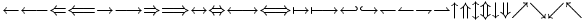(see also here)

## \Leftarrow (math symbol)

The \Leftarrow command is valid only in math mode. It generates an double-stroke arrow pointing to the left: <mo>&Leftarrow;</mo> (Unicode U+21D0, ⇐). See description of the \leftarrow command.

## \leftarrowtail (math symbol)

The \leftarrowtail command is valid only in math mode. It generates <mo>&leftarrowtail;</mo> (Unicode U+21A2, ↢)

## \leftarrowtriangle (math symbol)

The \leftarrowtriangle command is valid only in math mode. It generates <mo>&loarr;</mo> (Unicode U+21FD, ⇽).

## \leftharpoondown (math symbol)

The \leftharpoondown command is valid only in math mode. It generates an arrow that points to the left, with only lower part of the arrow-point: <mo>&leftharpoondown;</mo> (Unicode U+21BD, ↽). See description of the \leftarrow command.

## \leftharpoonup (math symbol)

The \leftharpoonup command is valid only in math mode. It generates an arrow pointing to the left, with only the upper part of the arrow-point: <mo>&leftharpoonup;</mo> (Unicode U+21BC, ↼). See description of the \leftarrow command.

## \lefthyphenmin (internal integer)

When you say \lefthyphenmin=77, then TeX accepts to hyphenate a word only if there are at least 77 characters to the left of the hyphen. Tralics hyphenates nothing.(See scanint for details of argument scanning).

## \leftleftarrows (math symbol)

The \leftleftarrows command is valid only in math mode. It generates <mo>&leftleftarrows;</mo> (Unicode U+21C7, ⇇)

## \leftline

Translation of \leftline{foo} is <line rend='left'>foo</line>.See also description of the \centerline command.

## \leftmargin (rigid length)

This contains the value of the left margin. Not used by Tralics.

## \leftmargini, \leftmarginii, \leftmarginiii, \leftmarginiv, \leftmarginv, \leftmarginvi (rigid length)

This command contains the value that should be put into \leftmargin inside a list environment (at level 1, 2, 3, 4, 5, and 6, respectively). Not used by Tralics.

## \leftrightarrow (math symbol)

The \leftrightarrow command is valid only in math mode. It generates an arrow that points to the left and the right: <mo>&leftrightarrow;</mo> (Unicode U+2194, ↔). See description of the \leftarrow command.

## \Leftrightarrow (math symbol)

The \Leftrightarrow command is valid only in math mode. It generates an arrow: <mo>&Leftrightarrow;</mo> (Unicode U+21D4, ⇔). See description of the \leftarrow command.

## \leftrightarrows (math symbol)

The \leftrightarrows command is valid only in math mode. It generates <mo>&leftrightarrows;</mo> (Unicode U+21C6, ⇆).

## \leftrightarrowtriangle (math symbol)

The \leftrightarrowtriangle command is valid only in math mode. It generates <mo>&hoarr;</mo> (Unicode U+21FF, ⇿).

## \leftrightharpoons (math symbol)

The \leftrightharpoons command is valid only in math mode. It generates <mo>&leftrightharpoons;</mo> (Unicode U+21CB, ⇋).

## \leftrightsquigarrow (math symbol)

The \leftrightsquigarrow command is valid only in math mode. It generates <mo>&leftrightsquigarrow;</mo> (Unicode U+21AD, ↭).

## \leftskip (rubber length)

You can say \leftskip=10pt plus 2pt minus 3pt. This explains to TeX that it should put 10pt (maybe up to 2pt more, maybe up to 3pt less) of glue on the start of each line. Unused by Tralics (you should use environments like quote or center instead).(See scanglue for details of argument scanning).

## \leftslice (math symbol)

The \leftslice command is valid only in math mode. It generates <mo>&#x2AA6;</mo> (Unicode U+2AA6, ⪦).

## \leftthreetimes (math symbol)

The \leftthreetimes command is valid only in math mode. It generates <mo>&leftthreetimes;</mo> (Unicode U+22CB, ⋋).

## \lengthtest

Inside an if-then-else construct, you can use a test like \lengthtest{\parindent<1cm}. This yields true if the current value of \parindent is less than one cm.See \ifthenelse for details.

## \leq (math symbol)

The \leq command is valid only in math mode. It is equivalent to \le. It generates a relation symbol: <mo>&le;</mo> (Unicode U+2264, ≤). See description of the \le command.

## \leqno

Produces an equation number on the left. See \eqno.

## \leqq (math symbol)

The \leqq is valid only in math mode; it produces <mo>&leqq;</mo> (Unicode U+2266, ≦).

## \leqslant (math symbol)

The \leqslant is valid only in math mode; it is a variant of the less-or-equal symbol: <mo>&leqslant;</mo> (Unicode U+2A7D, ⩽). See also description of the \smallint command.

## \lessapprox (math symbol)

The \lessapprox is valid only in math mode; it produces <mo>&lessapprox;</mo> (Unicode U+2A85, ⪅).

## \lessdot (math symbol)

The \lessdot is valid only in math mode; it produces <mo>&lessdot;</mo> (Unicode U+22D6, ⋖).

## \lesseqgtr (math symbol)

The \lesseqgtr is valid only in math mode; it produces <mo>&lesseqgtr;</mo> (Unicode U+22DA, ⋚).

## \lesseqqgtr (math symbol)

The \lesseqqgtr is valid only in math mode; it produces <mo>&lesseqqgtr;</mo> (Unicode U+2A8B, ⪋).

## \lessgtr (math symbol)

The \lessgtr is valid only in math mode; it produces <mo>&lessgtr;</mo> (Unicode U+2276, ≶).

## \lesssim (math symbol)

The \lesssim is valid only in math mode; it produces <mo>&lesssim;</mo> (Unicode U+2272, ≲).

## \let

After \let, there is a control sequence followed by equals followed by one optional space followed by token (see here the syntax). The effect of \let\foo\bar (or \let\foo= \bar) is to make \foo equivalent to \bar, concerning evaluation. Instead of \foo, an active character is allowed. If the token is a control sequence or an active character, its value is copied into the first token. For instance, since \bar is valid in math mode only, then \foo becomes a math-mode only command. An assignment like \let\foo\undefined makes probably \foo undefined. Example:

{\def\B#1{a} \let \A\B \show\A}
{\catcode A=13 \def A{b} \let\A A \show\A}
{\catcode A=13 \def\B#1{c} \let A\B \showA}
{\catcode A=13  \let A~ \showA}


You will see this on the terminal:

\A=macro: #1->a.
\A=macro: ->b.
A=macro: #1->c.
A=macro: ->\nobreakspace .


In the case where \let\foo is followed by a character, then \foo will act like this character (same catcode, same value). Example:

{\catcode A=13 \letA=1 \showA}
{\let\sp=^ \show\sp}
{\let\egroup=} \show\egroup}


You will see this:

A=the character 1.
\sp=superscript character ^.
\egroup=end-group character }.


Note the following facts: In the example above, A is an active character, but cannot be expanded. Thus \count0=0A1 will put 0 in the counter, and \showA is a list of two tokens. On the other hand, \sp is always interpreted as superscript character (but \sp\sp ab is not the same as ^^ab). And \egroup is evaluated like a closing brace (a token list contains always as many open braces as close braces, but you can put any number of \egroup tokens as you like; it is only when the token is evaluated that its value is considered; exception: when arguments are read, the value of each token is considered to see if it is an \outer token, so a token can be non-outer when read and outer when evaluated).You can say \global\let, this makes the assignment global (it is local otherwise) (unless \globaldefs is strange).## \lfloor (math symbol)

The \lfloor (left floor) command is valid only in math mode. It can be used as a delimiter <mo>&lfloor;</mo>, (Unicode U+230A, ⌊). See also description of the \vert command.

## \lfoot

The \lfoot command is valid only if the fancyvrb package is loaded. It takes an optional argument A, and a required argument B (the default value of A is B). The effect is to define the left part of the page footer to A for even pages, to B for odd pages.For more information, see documentation of \fancyhf.

## \lg (math symbol)

The \lg command is valid only in math mode. Its translation is a math operator of the same name: <mo form='prefix'>lg</mo>.For an example see the \log command.

## \lgroup (math symbol)

The \lgroup (left group) command is valid only in math mode. It can be used as a delimiter <mo>&#x3014;</mo>, (Unicode U+3014, 〔).

## \lhd (math symbol)

The \lhd command is valid only in math mode. It produces <mo>&#x22B2;</mo>, (Unicode U+22B2, ⊲).

## \lhead

The \lhead command is valid only if the fancyvrb package is loaded. It takes an optional argument A, and a required argument B (the default value of A is B). The effect is to define the left part of the page header to A for even pages, to B for odd pages.For more information, see documentation of \fancyhf.

## \lightning (math symbol)

The \lightning command is valid only in math mode. It produces <mo>&#x2607;</mo>, (Unicode U+2607, ☇).

## \lim (math symbol)

The \lim command is valid only in math mode. Its translation is a math operator of the same name: <mo form='prefix' movablelimits='true'>lim</mo>. For an example see the \log command.

## \liminf (math symbol)

The \liminf command is valid only in math mode. Its translation is a math operator of the same name: <mo form='prefix' movablelimits='true'>lim inf</mo>. For an example see the \log command.

## \limits

The \limits command is valid only in math mode. It describes how indices should be positioned. See \nolimits for details. For details see \mathord.

## \limsup (math symbol)

The \limsup command is valid only in math mode. Its translation is a math operator of the same name: <mo form='prefix' movablelimits='true'>lim sup</mo>. For an example see the \log command.

## \line

You say \line(dx,dy){length} in order to put a straight line into a picture. In general, the \line is the argument of a \put(x,y){} command, and the (x,y) position indicated the starting point of the line segment. Quantities dx and dy should be small integers (at most six in magnitude) and coprime (in fact, Tralics really does not care). If dx=0, then length is the length of the line; otherwise, length is the length of the horizontal projection of the line.See description of the picture environment for the syntax of the (dx,dy) part, and some examples.

## \linebreak

The \linebreak command takes an optional argument. It is used to favor a line break at this position in the paragraph. This command is ignored in Tralics.

## \linepenalty (internal integer)

When you say \linepenalty=13, then TeX will use 13 for the line penalty when it computes demerits. This parameter is not used in Tralics.(See scanint for details of argument scanning).

## \lineskip (rubber length)

You can say \lineskip=10pt plus 2pt minus 3pt. This explains to TeX that it should put 10pt (maybe up to 2pt more, maybe up to 3pt less) of glue between two consecutive lines in a paragraph. (if\lineskiplimit says so). Unused by Tralics.(See scanglue for details of argument scanning).

## \lineskiplimit (rigid length)

You can say \lineskiplimit=1.5in, after that TeX computes the vertical skip between two lines of text as follows: the baselines of the boxes are separated by \baselineskip, but is this would make the distance between the bottom of one box and the top of the other less than \lineskiplimit, then \lineskip is used as interline glue. Ignored by Tralics. Typical values in LaTeX are: \baselineskip=12pt, \lineskip=1pt, lineskiplimit=0pt.(See scandimen for details of argument scanning).

## \linethickness

The translation of \linethickness{0.7mm} could be <pic-linethickness size='0.7mm'/>.For an example of use, see \arc.

## \linewidth (rigid length)

This dimension is unused by Tralics. Initial value is 427pt in Tralics (nearly 15cm), the same as \textwidth.

## \listfiles

Using \listfiles has as side effect that, at the end of the run, Tralics will print some information about all files (gathered by \ProvidesClass, \ProvidesPackage and \ProvidesFile) to the terminal and transcript file, for instance

 *File List*
article.clt   2006/08/19 v1.0 article document class for Tralics
std.clt   2006/08/19 v1.0 Standard LaTeX document class, for Tralics
comma.plt   2007/12/29 v1.0 Insert commas every three digits (DPC)
checkend.plt   2007/12/14 v1.0 Checks for end environments
bbding.plt   2007/12/14 v1.0 Dingbats symbols
abstract.plt   2007/12/09 v1.1 configurable abstracts
keyval.plt   2007/12/08 v1.1 key=value parser for Tralics (DPC)
html.plt   2007/12/05 v1.0 Hypertext commands for latex2html
nopageno.plt   2007/12/31 v1.0 no page numbers
dummy.xml_in   2007/12/23 v1.0 Dummy file for Tralics
***********


## \listoffigures, \listoftables

These two commands terminate the current paragraph and insert a <listoffigures/> or a <listoftables/> element. For an example see \tableofcontents command.

## \listparindent (rubber length)

Quantity used by LaTeX, for paragraph indentation of all paragraphs in a list that do not start with \item; unused by Tralics.

## \ll (math symbol)

The \ll command is valid only in math mode. It generates a relation symbol: <mo>&ll;</mo> (Unicode U+226A, ≪). See description of the \le command.

## \llap

Translation of \llap{foo} is <line rend='llap'>foo</line>See also description of the \centerline command.

## \llbracket (math symbol)

The \llbracket command is valid only in math mode. Its translation is <mo>&LeftDoubleBracket;</mo>. (Unicode U+310A, 〚).

## \Lleftarrow (math symbol)

The \Lleftarrow command is valid only in math mode. It generates an arrow pointing to the left: <mo>&Lleftarrow;</mo>. (Unicode U+21DA, ⇚)

## \lll (math symbol)

The \lll command is valid only in math mode. Its translation is <mo>&Ll;</mo>. (Unicode U+22D8, ⋘).

## \llless (math symbol)

The \llless command is valid only in math mode. Its translation is <mo>&Ll;</mo>. (Unicode U+22D8, ⋘).

## \llparenthesis (math symbol)

The \llparenthesis command is valid only in math mode. Its translation is <mo>&#x2987;</mo>. (Unicode U+2987, ⦇).

## \lmoustache (math symbol)

The \lmoustache command is valid only in math mode. Its translation is <mo>&lmoustache;</mo>. (Unicode U+23B0, ⎰).

## \ln (math symbol)

The \ln command is valid only in math mode. Its translation is a math operator of the same name: <mo form='prefix'>ln</mo>.For an example see the \log command.

## \lnapprox (math symbol)

The \lnapprox command is valid only in math mode. Its translation is <mo>&lnapprox;</mo>. (Unicode U+2A89, ⪉).

## \lneq (math symbol)

The \lneq command is valid only in math mode. Its translation is <mo>&lneq;</mo>. (Unicode U+2A87, ⪇).

## \lneqq (math symbol)

The \lneqq command is valid only in math mode. Its translation is <mo>&lneqq;</mo>. (Unicode U+2268, ≨).

## \lnot (math symbol)

The \lnot command (logical not) is equivalent to \neg command. (Unicode U+AC, ¬).See also description of the \smallint command.

## \lnsim (math symbol)

The \lnsim command is valid only in math mode. Its translation is <mo>&lnsim;</mo>. (Unicode U+22E6, ⋦).

## \LoadClass

This is like \documentclass, except that in can be used anywhere (in general from a class file), can be used more than once, and unused options do not become global options.

## \LoadClassWithOptions

This is like \RequirePackageWithOptions, but is used in a class rather than a package. It loads the class file defined by the argument, using current options.

## \log (math symbol)

The \log command is valid only in math mode. Its translation is a math operator of the same name <mo form='prefix'>log</mo>. Here is the list of all log-like operators:


$$\arccos^2 (x),\, \arcsin^2(x),\, \arctan^2(x),\, \arg^2(x),\, \cos^2(x),\, \cosh^2(x) ,\, \cot^2(x)$$
$$\coth^2(x),\, \csc^2(x),\, \deg^2(x),\, \dim^2(x),\, \exp^2(x),\, \hom^2(x),\, \ker^2(x) ,\, \lg^2(x)$$
$$\ln^2(x),\, \log^2(x),\, \Pr^2(x),\, \sec^2(x),\, \sin^2(x) ,\,\sinh^2(x),\, \tan^2(x),\, \tanh^2(x)$$
$$%These are declared m \det_{x=1},\, \gcd_{x=1},\, \inf_{x=1},\, \injlim_{x=1},\, \lim_{x=1},\, \liminf_{x=1},\,\limsup_{x=1},\,\max_{x=1},\, \min_{x=1},\, \projlim_{x=1},\, \sup_{x=1}$$


The XML result is shown here. (see also here). All commands are listed in Table 8.9 of the Latex Companion.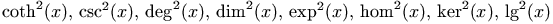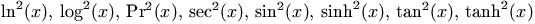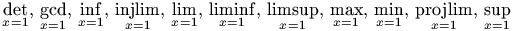## \loggingoutput

This command is ignored by Tralics; in LaTeX it is a piece of \tracingall, in particular it sets all parameters so that boxes are fully displayed when printed. You should say \tracingall in Tralics.

## \long

You can say \long\def\foo{...}. This defines the \foo command to be \long, said otherwise, \par tokens are allowed inside the argument. In the case of \newcommand\foo{...} the result is a \long command, and \newcommand*\foo{...} produces a non-\long one. A test done by \ifx is false if one command is \long and the other is not. In old versions Tralics, the behavior of the commands did not depend on the flag. If the source file contains

\def\tla#1{#1}
\long\def\tlb#1{#1}
\outer\def\tlc#1{#1}
\long\outer\def\tld#1{#1}
\newcommand\tle{#1}
\newcommand*\tlf{#1}
\show\tla \show\tlb \show\tlc \show\tld \show\tle \show\tlf


then Tralics will print this

\tla=macro: #1->#1.
\tlb=\long macro: #1->#1.
\tlc=\outer macro: #1->#1.
\tld=\long\outer macro: #1->#1.
\tle=\long macro: #1->#1.
\tlf=macro: #1->#1.


The next example shows the difference between a \long and a non-\long command.

> \newcommand\foo{} \foo{\par}
> \renewcommand*\foo{} \foo{\par}
Error signaled at line 5:
Runaway argument?
Paragraph ended while scanning an argument of \foo.


## \longleftarrow (math symbol)

The \longleftarrow command is valid only in math mode. It generates an arrow pointing to the left: <mo>&longleftarrow;</mo> (Unicode U+27F5, ⟵). See description of the \leftarrow command.

## \Longleftarrow (math symbol)

The \Longleftarrow command is valid only in math mode. It generates a double stroke long arrow pointing to the left: <mo>&Longleftarrow;</mo> (Unicode U+27F8, ⟸). See description of the \leftarrow command.

## \longleftrightarrow (math symbol)

The \longleftrightarrow command is valid only in math mode. It generates an arrow pointing to the left and the right: <mo>&longleftrightarrow;</mo> (Unicode U+27F7, ⟷). See description of the \leftarrow command.

## \Longleftrightarrow (math symbol)

The \Longleftrightarrow command is valid only in math mode. It generates a long doublestroke arrow pointing to the left and the right: <mo>&Longleftrightarrow;</mo> (Unicode U+27FA, ⟺). See description of the \leftarrow command.

## \longmapsfrom (math symbol)

The \longmapsfrom command is valid only in math mode. It generates an arrow: <mo>&#x27FB</mo> (Unicode U+27FB, ⟻).

## \Longmapsfrom (math symbol)

The \Longmapsfrom command is valid only in math mode. It generates an arrow: <mo>&#x27FD;</mo> (Unicode U+27FD, ⟽).

## \Longmapsto (math symbol)

The \Longmapsto command is valid only in math mode. It generates an arrow: <mo>&#x27FE;</mo> (Unicode U+27FE, ⟾).

## \longmapsto (math symbol)

The \longmapsto command is valid only in math mode. It generates an arrow: <mo>&longmapsto;</mo> (Unicode U+27FC, ⟼). See description of the \leftarrow command.

## \longrightarrow (math symbol)

The \longrightarrow command is valid only in math mode. It generates a long arrow pointing to the right: <mo>&longrightarrow;</mo> (Unicode U+27F6, ⟶). See description of the \leftarrow command.

## \Longrightarrow (math symbol)

The \Longrightarrow command is valid only in math mode. It generates a long double stroke arrow pointing to the right: <mo>&Longrightarrow;</mo> (Unicode U+27F9, ⟹) See description of the \leftarrow command.

## \loop

The \loop command can be defined as

% Plain tex definition
\def\loop#1\repeat{\def\body{#1}\iterate}
\def\iterate{\body \let\next\iterate \else\let\next\relax\fi \next}
\let\repeat=\fi % this makes \loop...\if...\repeat skippable
% Latex definition
\def\loop#1\repeat{\def\iterate{#1\relax\expandafter\iterate\fi}%
\iterate \let\iterate\relax}
\let\repeat\fi


The definition of Tralics is the same as the definition of LaTeX, but the \iterate token used is private (different from all commands that the user can enter).

You say \loop alpha \if... beta \repeat, where alpha and beta are any sequences of commands (if they contain a \repeat token, it must be hidden in braces) and where \if... is any conditional test, without a matching \fi. TeX will first do alpha, then if the condition is true, TeX will do beta and repeat the whole process again starting with alpha. If the condition ever turns out to be false, the loop will stop.

Consider

\def\looptest#1#2#3{\count0=#1\relax
\loop #2\advance\count0by-1
\ifnum\count0>0 #3\repeat}


Here the first argument of \looptest will be put in count register 0, the third argument is beta, the condition is \ifnum\count0>0 the alpha is formed of the second argument and a piece of code that decrements the counter. Said otherwise, if the first argument is 5, then the second argument will be executed 5 times, and the third argument 4 times. Example:

\def\looptest#1#2#3{\count0=#1\relax \loop #2\advance\count0by-1
\ifnum\count0>0 #3\repeat}
\def\res{}
\looptest5{\edef\res{\the\count0 a\res}}{\edef\res{b\res}}
\testeq\res{1ab2ab3ab4ab5a}%The command \testeq checks that its arguments are equalSee also commented example.

## \looparrowleft (math symbol)

The \looparrowleft command is valid only in math mode. It generates <mo>&looparrowleft;</mo> (Unicode U+21AB, ↫).

## \looparrowright (math symbol)

The \looparrowright command is valid only in math mode. It generates <mo>&looparrowright;</mo> (Unicode U+21AC, ↬).

## \looseness (internal integer)

When you say \looseness=90, then TeX will try to make the current paragraph 90 lines longer than its optimum length; the badness of each individual line cannot exceed the given tolerance. Unused by Tralics.(See scanint for details of argument scanning).

## \lor (math symbol)

The \lor command (logical or) is equivalent to \vee command: <mo>&vee;</mo> (Unicode U+2228, ∨). See also description of the \smallint command.

## \lower

This lowers a box. See \moveleft.

## \lowercase

The \lowercase command converts its argument to lowercase. For instance \lowercase{Foo+\Bar} is equivalent to foo+\Bar. (See \uppercase.) (see scantoks for the syntax.)

## \lozenge

The \lozenge command is valid in math mode only; it produces the symbol <mo>&lozenge;</mo> (Unicode U+25CA, ◊).

## \lq

The \lq command expands to a left quote (back-quote). You can say \lq\foo\rq' or `\foo'', the result is the same.

## \lsc

This command is defined by compatibility with the french package. The translation of \lsc{Abc} is <hi rend='sc'>Abc</hi>

## \Lsh

The \Lsh command is valid in math mode only; it produces the symbol <mo>&Lsh;</mo> (Unicode U+21B0, ↰).

## \ltimes

The \ltimes command is valid in math mode only; it produces the symbol <mo>&ltimes;</mo> (Unicode U+22C9, ⋉).

## \lvert (math symbol)

The \lvert command is valid in math mode only; it is a left delimiter associated to \vert; it produces the symbol <mo>&vert;</mo> (Unicode U+7C, |).

## \lVert (math symbol)

The \lVert command is valid in math mode only; it is a left delimiter associated to \Vert; it produces the symbol <mo>&parallel;</mo> (Unicode U+2225, ∥).back to home page © INRIA 2003-2005, 2006 Last modified $Date: 2015/12/01 17:24:42$